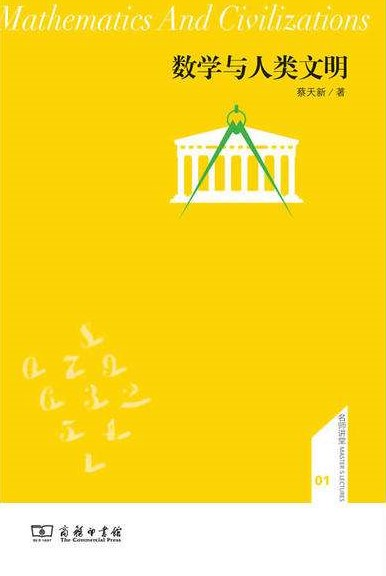It looks like you're using Internet Explorer 11 or older. This website works best with modern browsers such as the latest versions of Chrome, Firefox, Safari, and Edge. If you continue with this browser, you may see unexpected results.

# GEB2402 A Journey to Mathematics: Home

## Course Description

This course is not intended to teach students any advanced mathematical skills in solving complicated equations. As a general education course, it aims at providing students with a brief overview of mathematics in an understandable manner. Emphasis will be put on the influences of mathematics on our culture, society, science, technology and various human activities, e.g. abstract thinking, philosophy, politics, management, language, art etc.

## Recommended Books### Journey through Genius: The Great Theorems of Mathematics

This book is written for general readers who are interested in the history of mathematics. It provides a journey through centuries of achievements in the field of mathematics. This book not only focuses on the theorem per se, but also cares about the historical context of these theorems. Besides, this book presents a biographical feature- each theorem is associated with the mathematician that contributed to its development. In addition to the biographical and historical anecdotes the author uses algebra, geometry, and some trigonometry and calculus to show the reader exactly how the discoveries were made. Chapters are developed in chronological order.### The Mathematical Universe: An Alphabetical Journey through the Great Proofs, Problems, and Personalities

This book is an A-Z collection of essays that surveys the discipline of mathematics. It contains 25 alphabetical entries, and these entries cover fundamental theorems such as Arithmetic and Calculus, prolific mathematicians such as Euler, functionality of mathematics such as the process of mathematical justification, as well as contemporary social issues such as multiculturalism and gender equality, etc. The mathematical ideas and the people behind them are all presented in the historical context. It is primarily aimed at general readers at an introductory level. Nevertheless, professionals can find material of interest pertaining to areas removed from their specialties.### Mathematics: A Very Short Introduction

This volume on Mathematics is aimed at general readers, and thus requires little prior knowledge. The abstract ideas and concepts presented in the book enable a reader to go through and understand the mathematical structures and patterns. There are eight chapters, covering the building of mathematical models; numbers and further expands on abstraction; the mathematical proofs mathematicians and scientists develop on the bases of axioms; the concepts of limits and infinities; the concept of dimensions; geometry; the estimates and approximations; as well as common sociological questions about the mathematical community.### The Story of Mathematics

This book is a concise survey of the history of mathematics. The goal of the book is "to illustrate how the mathematical sciences were intimately linked to the interests and aspirations of the civilizations in which they flourished," which is achieved in a fast panorama virtually without equations or mathematical symbolism. The text travels from the dawn of Chinese and Indian civilizations to the scientific and digital revolutions of our day. The content covers astronomy, ancient and medieval mathematics from various cultures, Newton, cartography, the quintic, noneuclidean geometry, infinity, chance, game theory, noneuclidean dimensions of modern art, computing, and chaos/fractals, etc.### The Book of Numbers

This book surveys number names, words and symbols and explains the new phenomenon of surreal numbers. Chapter 1 describes number words and symbols. Chapter 2 show how many elementary but important facts can be discovered without using any mathematics. Chapter 3 and 4 exhibit several sets of whole numbers which can manifest themselves in quite different contexts. Chapter 5 is devoted to the prime numbers. Chapters 6 and 7 deal with fractions. Chapters 8 and 9 explains “complex” and “transcendental” numbers. Chapter 10 is devoted to the infinite and the infinitesimal, to surreal numbers.### 数学与现代生活